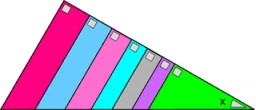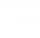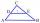# Sin cos tan

In triangle ABC, right-angled at B.
Sides/AB/=7cm, /BC/=5cm, /AC/=8.6cm.
Find to two decimal places.
A. Sine C
B. Cosine C
C. Tangent C.

S =  0.814
K =  0.5814
T =  1.4

### Step-by-step explanation:Did you find an error or inaccuracy? Feel free to write us. Thank you!Tips to related online calculators
Most natural application of trigonometry and trigonometric functions is a calculation of the triangles. Common and less common calculations of different types of triangles offers our triangle calculator. Word trigonometry comes from Greek and literally means triangle calculation.

#### You need to know the following knowledge to solve this word math problem:

We encourage you to watch this tutorial video on this math problem:

## Related math problems and questions:

• Parallelogram ABCDWe have the parallelogram ABCD, where AB is 6.2 cm BC is 5.4 cm AC is 4.8 cm calculate the height on the AB side and the angle DAB
• Right triangleCalculate the length of the remaining two sides and the angles in the rectangular triangle ABC if a = 10 cm, angle alpha = 18°40'.
• The right triangleIn the right triangle ABC with a right angle at C, we know the side lengths AC = 9 cm and BC = 7 cm. Calculate the length of the remaining side of the triangle and the size of all angles.
• Trigonometric functionsIn the right triangle is: tg α= frac(2) 1 Find the value of s and c: sin α= (s)/(√ 5) cos α= (c)/(√ 5)
• SinesIn ▵ ABC, if sin(α)=0.5 and sin(β)=0.6 calculate sin(γ)
• Right triangle trigonometricsCalculate the size of the remaining sides and angles of a right triangle ABC if it is given: b = 10 cm; c = 20 cm; angle alpha = 60° and the angle beta = 30° (use the Pythagorean theorem and functions sine, cosine, tangent, cotangent)
• Right triangleDraw a right triangle ABC if |AB| = 5 cm |BC| = 3 cm, |AC| = 4 cm. Draw Thales circle above the hypotenuse of the triangle ABC.
• Trapezoid IVIn a trapezoid ABCD (AB||CD) is |AB| = 15cm |CD| = 7 cm, |AC| = 12 cm, AC is perpendicular to BC. What area has a trapezoid ABCD?
• Right angled triangle 3Side b = 1.5, hypotenuse angle A = 70 degrees, Angle B = 20 degrees. Find its unknown sides length.
• Right angleIn a right triangle ABC with a right angle at the apex C, we know the side length AB = 24 cm and the angle at the vertex B = 71°. Calculate the length of the legs of the triangle.
• Squares above sidesTwo squares are constructed on two sides of the ABC triangle. The square area above the BC side is 25 cm2. The height vc to the side AB is 3 cm long. The heel P of height vc divides the AB side in a 2: 1 ratio. The AC side is longer than the BC side. Calc
• Triangle ABC v2Area of the triangle is 12 cm square. Angle ACB = 30º , AC = (x + 2) cm, BC = x cm. Calculate the value of x.Calculate the content of a regular 15-sides polygon inscribed in a circle with radius r = 4. Express the result to two decimal places.A right triangle ABC is given, c is a hypotenuse. Find the length of the sides a, b, the angle beta if c = 5 and angle alfa = A = 35 degrees.If tg α = 9.6, Calculating sin α, cos α, cotg α .In a triangle ABC with the side/AB/ = 24 cm is constructed middle segment/DE/ = 18 cm parallel to the side AB at a distance 1 cm from AB. Calculate the height of the triangle ABC to side AB.| AB | = 76cm, | BC | = 44cm, angle BAD = 30 °. Find the area of the parallelogram.